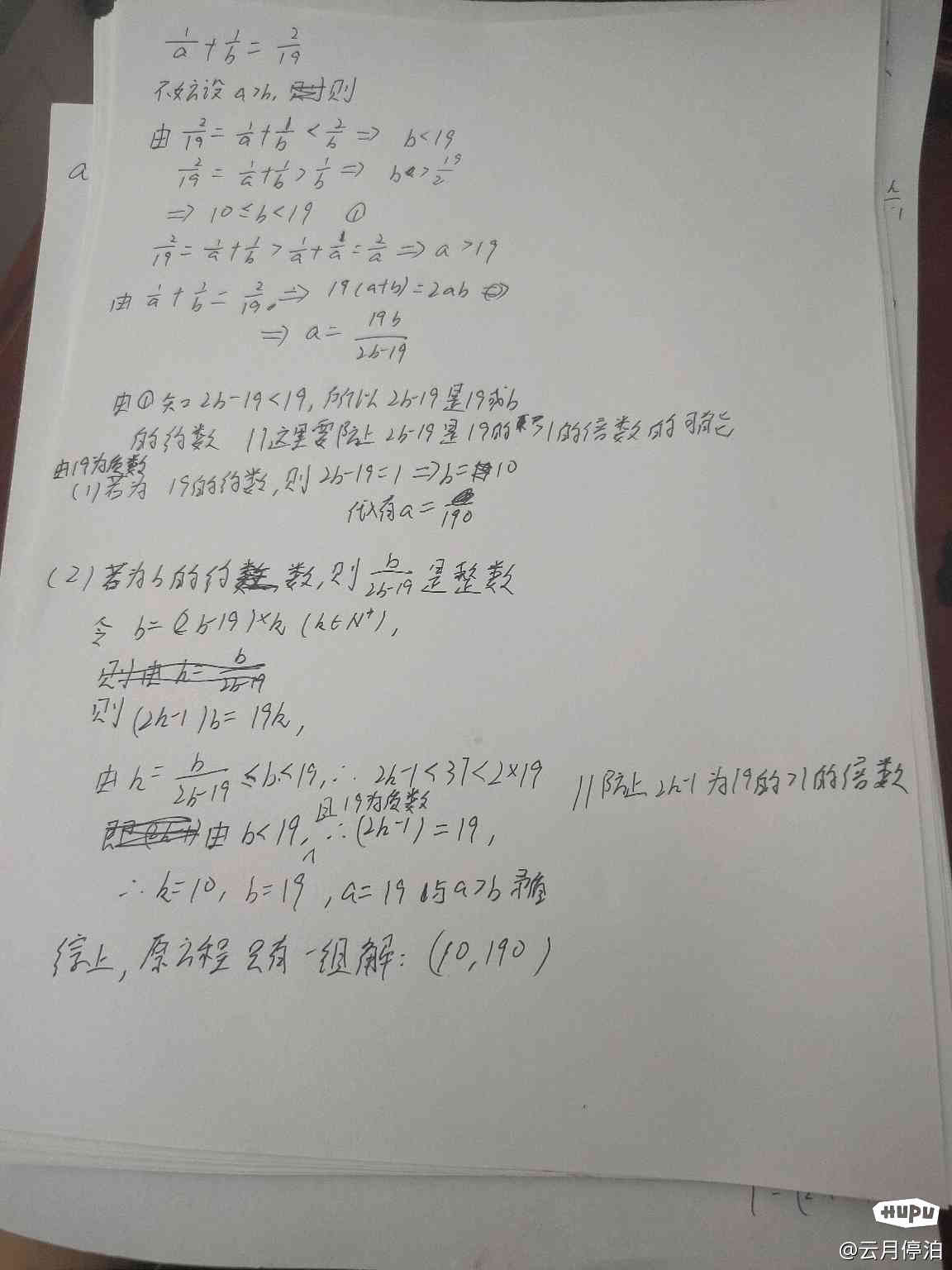NBA英雄

NBA范特西

# 救命啊！小学题都这么难了？

108回复/ 24381215 浏览10和190

10和190

10和190

10和19010和190

10和19010和190

10和190

“不同”

“不同”

[图片][图片][图片]“填入 不同的 自然数”

“填入 不同的 自然数”10和190

10和190

“不同”

“不同”

1/X+1/Y=1/19有个非常简便的算法，X=19+1=20，Y=19*20=380。

1/X+1/Y=1/19有个非常简便的算法，X=19+1=20，Y=19*20=380。

X=（15+1）/2=8。
Y=（15*（15+1））/2=120。

X=（15+1）/2=8。
Y=（15*（15+1））/2=120。

10和190

10和1901/N=1/（N+1）+1/（N*（N+1））

1/N=1/（N+1）+1/（N*（N+1））

1/N=1/（N+1）+1/（N*（N+1））

👍🏻

👍🏻

19n=（2n-1）a。

19n=（2n-1）a。

19n=（2n-1）a。

3*45n=（2n-1）a
9*15n=（2n-1）a
27*5n=（2n-1）a
135n=（2n-1）a

19n=（2n-1）a。

3*45n=（2n-1）a
9*15n=（2n-1）a
27*5n=（2n-1）a
135n=（2n-1）a

Re：救命啊！小学题都这么难了？